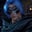Related Tags

python3
java
communitycreator

# How to interchange the first and last elements in a listGutha Vamsi Krishna

### Problem

Given a list, swap the first and the last elements of the list.

### Example

Input: [23, 100, 54, 56, 98]

Output: [98, 100, 54, 56, 23]

### Python solution

We will use a Python feature to solve this which assigns multiple variables at the same time.

For example, if we have variables a and b, then we can assign values to it like this:

a,b = 10,20


Where:

10 is stored in a, and 20 is stored in b.

### Code

#list
lst = [23, 100, 54, 56, 98]

#interchanging the first and last element
lst, lst[-1] = lst[-1], lst

print(lst)

### Explanation

In the following code snippet:

• Line 2: We initialize lst with [23, 100, 54, 56, 98].
• Line 5: We use the Python feature mentioned above to interchange the first and last elements in the list, with lst, lst[-1] = lst[-1], lst, where with lst[-1], we can access last element.
• Line 7: We print the list where the first and last elements are interchanged.

### Java solution

We will use a temporary variable to interchange the first and last elements in a list.

• First, we will store the first element in a temporary variable.
• Then, we assign the last element to the first element.
• Lastly, we assign a temporary variable to the last element.

### Code

import java.util.*;

class HelloWorld {
public static void main( String args[] ) {
//initialize the list
int[] arr = {23, 100, 54, 56, 98};
//temp variable
int temp = arr;
arr = arr[arr.length-1];
arr[arr.length-1] = temp;
//printing modified array
System.out.println(Arrays.toString(arr));
}
}

### Explanation

In the following code snippet:

• We initialize array arr with {23, 100, 54, 56, 98}.
• We store the first element arr in temp.
• Now, we assign the last element to the first element, and we get the last element by subtracting 1 from the array length.
• Now, we assign temp to the last element.

RELATED TAGS

python3
java
communitycreator

CONTRIBUTORGutha Vamsi Krishna
RELATED COURSES

View all Courses

Keep Exploring

Learn in-demand tech skills in half the time